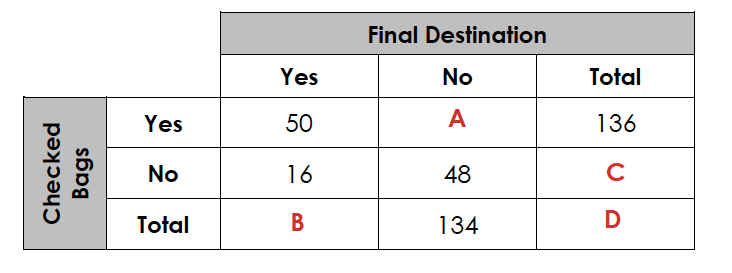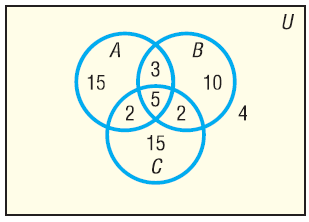Simple Probability
Compound Probability
Two-Way Tables
Venn Diagrams
100

What is the probability of rolling a 4 with a 6 sided die?

1/6

0.1667

16.67%

100

A coin is tossed. What is the probability of flipping heads two times in a row?

1/4

0.25

25%

100

What value goes in the space labeled "C"?16 + 48 = 64

100In the Venn Diagram above, how many are in set A?

25

200

What is the probability of drawing the queen of hearts from a deck of cards?

1/52

0.019

1.9%

200
A coin is tossed then a 6-sided die is rolled. What is the probability of flipping heads then rolling a 4?

1/12

0.083

8.3%

200

What value goes in the space labeled "A"?136 - 50 = 86

200In the Venn Diagram above, how many are in set A or B?

37

300

What is the probability of drawing a 5 or 6 from a deck of cards?

8/52 or 2/13

0.154

15.4%

300

A bag contains 3 red, 5 blue, 6 green, and 6 yellow marbles. What is the probability of drawing a blue marble then red marble if the marbles are put back each time.

5/20 x 3/20 = 15/400 or 3/80

0.0375

3.75%

300

What is the probability that someone in class A passed the test?19/22

0.863

86.3%

300In the Venn Diagram above, how many are in set A or B but not C?

28

400

A bag contains 3 red, 5 blue, 6 green, and 6 yellow marbles. What is the probability of drawing a blue marble?

5/20 or 1/4

0.25

25%

400

A bag contains 3 red, 5 blue, 6 green, and 6 yellow marbles. What is the probability of drawing a yellow marble then blue marble if the marbles are NOT put back each time.

6/20 x 5/19 = 30/380 or 3/38

0.0789

7.89%

400

What is the P(in class A or Passed)in class A or passed = 19+24+21+3 = 67

total students = 80

67/80

0.8375

83.75%

400

Draw a Venn Diagram then answer the following question:

In a survey of 100 people, 40 owned a cat, 50 owned a dog, 25 owned another kind of pet ("other"), 25 owned a cat and a dog, 10 owned a cat and another kind of pet, 15 owned a dog and another kind of pet, and 5 owned all three. How many people in the survey did not own a dog, cat, or another kind of pet?

30

500

A bag contains 3 red, 5 blue, 6 green, and 6 yellow marbles. What is the probability of drawing a blue or red marble?

8/20 or 2/5

0.4

40%

500

A coin is flipped 5 times. What is the probability of getting heads each time?

1/2 \times1/2\times 1/2 \times1/2\times 1/2 = 1/32

=0.03125

=3.125%

500

What is the P(Did not Pass | Class C)5/26

0.192

19.2%

500

Draw a Venn Diagram and answer the following question:

In a survey of 100 people, 40 owned a cat, 50 owned a dog, 25 owned another kind of pet ("other"), 25 owned a cat and a dog, 10 owned a cat and another kind of pet, 15 owned a dog and another kind of pet, and 5 owned all three. How many people in the survey owned a dog or a cat, but not another kind of pet?

45

Click to zoom